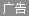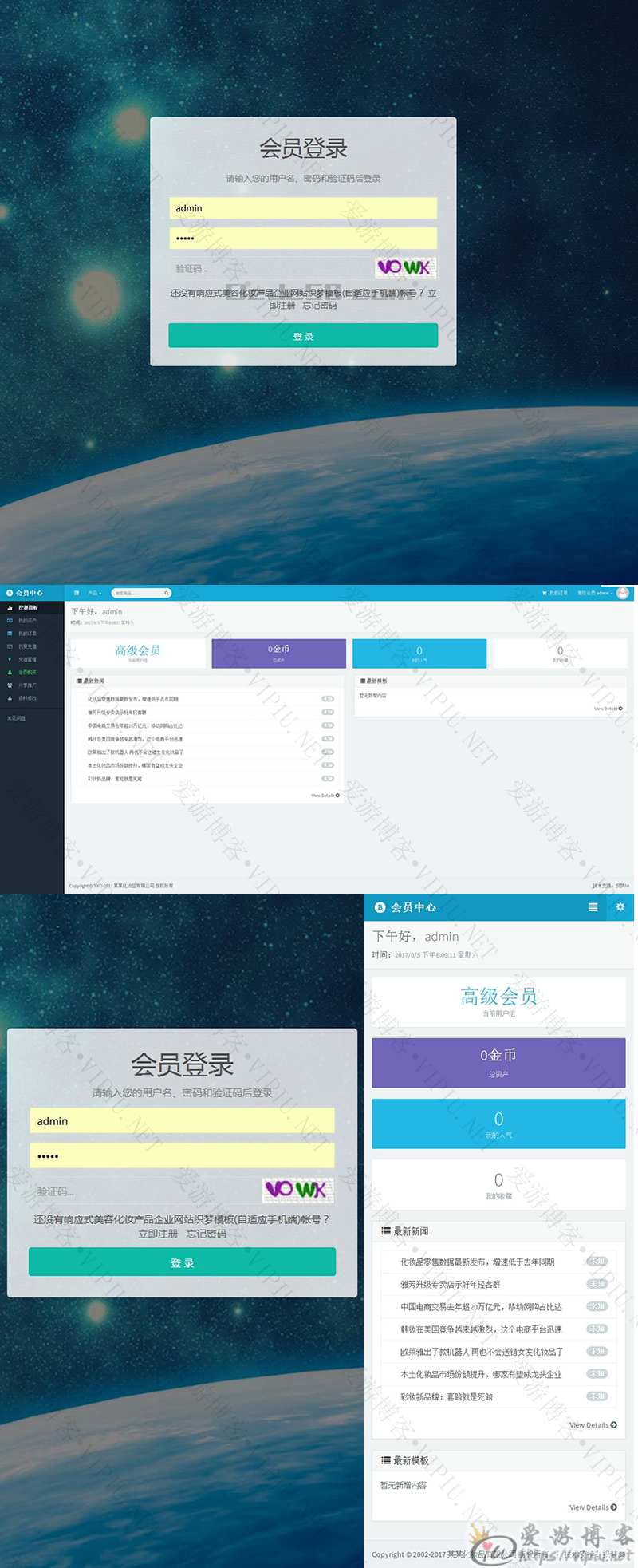# [织梦模板]响应式自适应手机端会员中心UTF-8及GBK编码1、将 user 文件夹上传至网站根目录

2、修改 include 文件夹下 common.inc.php 文件

`define('DEDEMEMBER', DEDEROOT.'/member');`

`define('DEDEMEMBER', DEDEROOT.'/user');`

//会员目录

```\$cfg_member_dir = \$cfg_cmspath.'/member';
\$cfg_memberurl = \$cfg_mainsite.\$cfg_member_dir;```

//会员目录

```\$cfg_member_dir = \$cfg_cmspath.'/user';
\$cfg_memberurl = \$cfg_mainsite.\$cfg_user_dir;```

3、首页最新新闻与最新模板修改方法

```WHERE typeid=12 order BY arc.id DESC LIMIT 0, 8 ";
typeid=12 代表栏目ID， arc.id DESC LIMIT 0, 8 显示8条信息```

4、财付通、支付宝默认收款账号修改

```<?php
\$a1=\$_POST['a1'];     //用户名
\$cny=\$_POST['cny'];  //充值金额
\$a3=\$_POST['a3'];   //充值方式

\$pay1="meike203@qq.com";//支付宝收款账号
\$pay2="970003436";//财付通收款账号

//-------------支付宝跳转开始
if(\$a3==1){
?>```